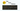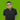# Map, Filter, Reduce - Code ExercisesWritten by David Abram

It's really hard to test your programming knowledge after you have completed a tutorial or some lecture. We have prepared some exercises to help out the beginner devs to solidify their understanding of map, filter, reduce, and other useful array methods. Every exercise has a brief description of the problem, starting code, links to relevant MDN docs, and expected results. Try to solve the problems without taking a peek at the solution.

If you need some additional help, you can check out our Arrays video from #lockdown learning series in which we discuss map, filter and reduce or contact the author of the article directly.

As a JavaScript developer, you will come across arrays. Arrays of numbers, arrays of objects, arrays of arrays of objects, multi-dimensional arrays, and you will need to simplify, transform or manipulate them.

You could use nested for loops to solve most of the problems you will encounter, but that leaves the code hard to read and understand.
I would like to prove to you that by using map, filter, and reduce array methods, not only that the code will be more readable, but you will be able to analyze the problem better and write the code with ease.

## Array Squared

Square value of every element in the array. Presume that you will get only numbers in the input array.

#### Input

``  const input = [ 1, 2, 3, 4, 5 ];``

#### Result

``  [ 1, 4, 9, 16, 25]``

#### Solution

(click to show)
``````  const input = [ 1, 2, 3, 4, 5 ];

input.map(function(num) {
return Math.pow(num, 2);
});

// or written with Arrow function
input.map(num => Math.pow(num, 2));``````

## Sum of every positive element

Input is an array of numbers, return the sum of all of the positives ones. If the array is empty or there aren't any positive numbers return 0.

#### Input

``  const input = [ 1, -4, 12, 0, -3, 29, -150];``

#### Result

``  42``

#### Solution

(click to show)
``````  const input = [ 1, -4, 12, 0, -3, 29, -150];

input
.filter(function(num) {
return num > 0;
})
.reduce(function(accumulator, currentValue) {
return accumulator + currentValue;
}, 0);

// or written with Arrow function
input.filter(num => num > 0)
.reduce((accumulator, currentValue) => accumulator + currentValue, 0);``````

## Calculate median and mean

Calculate the mean and median values of the number elements from the input array.

#### Input

``````  const input = [12, 46, 32, 64];
``````

#### Result

``  { mean: 38.5, median: 32 }``

#### Solution

(click to show)
``````  const input = [12, 46, 32, 64];
input.sort((a, b) => a - b);

input
.reduce((accumulator, currentValue, index, array) => {

accumulator.mean += currentValue /  array.length;

if(Math.abs(index + 1  - array.length / 2) < 1) {
accumulator.median = currentValue
}

return accumulator;
}, { mean: 0, median: 0 });``````

## Get Name initials

Input is a string of multiple words with a single space between each of them. You should abbreviate the name and get the name initials.

#### Input

``  const input = 'George Raymond Richard Martin';``

#### Result

``  'GRRM'``

#### Solution

(click to show)
``````  const input = 'George Raymond Richard Martin';

input
.split(' ')
.map(function(word) {
return word;
})
.join('');

// or written with Arrow function
input.split(' ').map(word => word).join('');``````

## Age Difference from the youngest and oldest

Find the difference in age between the oldest and youngest family members, and return their respective ages and the difference.

#### Input

``````  const input = [
{
name: 'John',
age: 13
},
{
name: 'Mark',
age: 56,
},
{
name: 'Rachel',
age: 45,
},
{
name: 'Nate',
age: 67,
},
{
name: 'Jeniffer',
age: 65,
}
];
``````

#### Result

``  [13, 67, 54]``

#### Solution

(click to show)
``````  const input = [
{
name: 'John',
age: 13
},
{
name: 'Mark',
age: 56,
},
{
name: 'Rachel',
age: 45,
},
{
name: 'Nate',
age: 67,
},
{
name: 'Jeniffer',
age: 65,
}
];

const ages = input.map(person => person.age);

[Math.min(...ages), Math.max(...ages), Math.max(...ages) - Math.min(...ages)];``````

## Numeronyms

Devs like to abbreviate everything: k8s means Kubernetes, a11y means accessibility, l10n means localization. You get the Dev numeronyms by taking the first and the last letter and counting the number of letters in between. Words that have less than 4 letters aren't abbreviated, because that would be just odd. The input is a sentence, and you should abbreviate every word that is 4 letters long or longer. There won't be any punctuation in the sentence. g2d l2k e6e

#### Input

``  const input = 'Every developer likes to mix kubernetes and javascript';``

#### Result

``  'E3y d7r l3s to mix k8s and j8t'``

#### Solution

(click to show)
``````  const input = 'Every developer likes to mix kubernetes and javascript';

const createNumeronym = (word) => word + (word.length - 2) +  word[word.length - 1];

input
.split(' ')
.map(function(word) {
if(word.length >= 4) {
return createNumeronym(word);
}
return word;
})
.join(' ');

// or written with Arrow function and Conditional operator
input
.split(' ')
.map(word => word.length >= 4 ? createNumeronym(word) : word)
.join(' ');``````

## n! with map and reduce

Input is a number and you should return the factorial of that number. The factorial of a natural number n is the product of the positive integers less than or equal to n. So, 2! = 2, 3! = 6, 4! = 24 and so on.

#### Input

``  const input = 6;``

#### Result

``  720``

#### Solution

(click to show)
``````  const input = 6;

const array = new Array(input).fill(null);
// array is [null, null, null, null, null, null]

array
.map(function(currentValue, index) {
return index + 1;
})
.reduce(function(accumulator, currentValue) {
return accumulator * currentValue;
});

// or written with Arrow function
new Array(input)
.fill(null)
.map((currentValue, index) => index + 1)
.reduce((accumulator, currentValue) => accumulator * currentValue);``````

## Count elements in array of arrays

Count the occurrences of distinct elements in the given 2D array. The given input is an array in which elements are arrays of strings. A result is an object which properties' names are values from the arrays and their value is the number of their occurrences.

#### Input

``````  const input = [
['a', 'b', 'c'],
['c', 'd', 'f'],
['d', 'f', 'g'],
];``````

#### Result

``````  {
a: 1,
b: 1,
c: 2,
d: 2,
f: 2,
g: 1,
}``````

#### Solution

(click to show)
``````  const input = [
['a', 'b', 'c'],
['c', 'd', 'f'],
['d', 'f', 'g'],
];

input
.flat()
.reduce((accumulator, currentValue) => {
if(accumulator[currentValue]) {
accumulator[currentValue] += 1;
} else {
accumulator[currentValue] = 1;
}
return accumulator;
}, {});``````David Abram
Spends his time untangling software architectures and doing DevOps. Likes to build stuff. Connect with him on Twitter and LinkedIn